Next: Venus Up: The Inferior Planets Previous: The Inferior Planets

# Determination of Ecliptic Longitude

Figure 33 compares and contrasts heliocentric and geocentric models of the motion of an inferior planet (i.e., a planet which is closer to the sun than the earth),, as seen from the earth,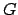. The sun is at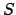. As before, in the heliocentric model the earth-planet displacement vector,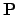, is the sum of the earth-sun displacement vector,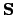, and the sun-planet displacement vector,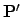. On the other hand, in the geocentric modelgives the displacement of the guide-point,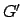, from the earth. Sinceis also the displacement of the sun,, from the earth,, it is clear thatexecutes a Keplerian orbit about the earth whose elements are the same as those of the apparent orbit of the sun about the earth. This implies that the sun is coincident with. The ellipse traced out byis termed the deferent. The vectorgives the displacement of the planet,, from the guide-point,. Sinceis also the displacement of the planet,, from the sun,, it is clear thatexecutes a Keplerian orbit about the guide-point whose elements are the same as those of the orbit of the planet about the sun. The ellipse traced out byaboutis termed the epicycle.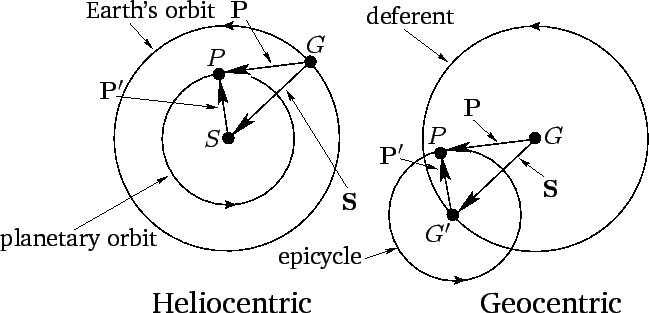As we have seen, the deferent of a superior planet has the same elements as the planet's orbit about the sun, whereas the epicycle has the same elements as the sun's apparent orbit about the earth. On the other hand, the deferent of an inferior planet has the same elements as the sun's apparent orbit about the earth, whereas the epicycle has the same elements as the planet's orbit about the sun. It follows that we can formulate a procedure for determining the ecliptic longitude of an inferior planet by simply taking the procedure used in the previous section for determining the ecliptic longitude of a superior planet and exchanging the roles of the sun and the planet.

Our procedure is described below. As before, it is assumed that the ecliptic longitude,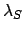, and the radial anomaly,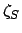, of the sun have already been calculated. In the following,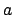,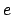,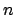,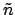,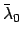, and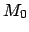represent elements of the orbit of the planet in question about the sun, whereas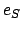is the eccentricity of the sun's apparent orbit about the earth. Again,is the major radius of the planetary orbit in units in which the major radius of the sun's apparent orbit about the earth is unity. The requisite elements for all of the inferior planets at the J2000 epoch (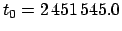JD) are listed in Table 30. The ecliptic longitude of an inferior planet is specified by the following formulae: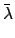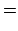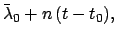(188)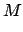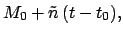(189)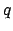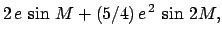(190)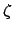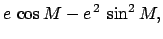(191)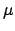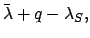(192)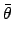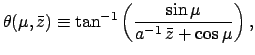(193)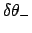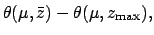(194)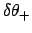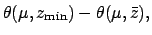(195)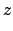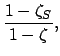(196)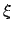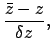(197)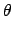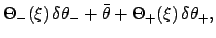(198)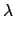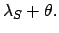(199)

Here,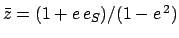,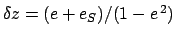,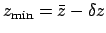, and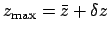. The constants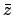,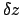,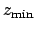, and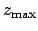for each of the inferior planets are listed in Table. 5. Finally, the functions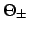are tabulated in Table 17.

For the case of Venus, the above formulae are capable of matching NASA ephemeris data during the years 1995-2006 CE with a mean error of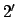and a maximum error of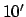. For the case of Mercury, given its relatively large eccentricity of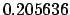, it is necessary to modify the formulae slightly by expressingandto third-order in the eccentricity: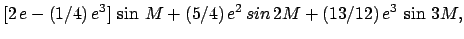(200)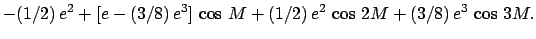(201)

With this modification, the mean error is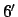and the maximum error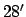.Next: Venus Up: The Inferior Planets Previous: The Inferior Planets
Richard Fitzpatrick 2010-07-21